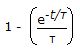# Chemical Engineering - Chemical Reaction Engineering

Exercise : Chemical Reaction Engineering - Section 8
1.
For the same residence time, which one will give the maximum conversion ?
Single stirred tank (v = 5 litres).
Two stirred tank (each of 2.5 litres) in series.
Stirred tank followed by tubular flow reactor (each of 2.5 litres).
Single tubular flow reactor (v = 5 litres).
Explanation:
No answer description is available. Let's discuss.

2.
__________ gas is normally employed in B.E.T.method of finding out the surface area of catalyst.
N2
H2
CO2
He
Explanation:
No answer description is available. Let's discuss.

3.
The exit age distribution curve E(t) for an ideal CSTR with the average residence time, τ, is given by
e-t/τ1 - e-t/τExplanation:
No answer description is available. Let's discuss.

4.
The rate constant of a reaction depends on the
initial concentration of reactants.
time of reaction.
temperature of the system.
extent of reaction.
For the reversible reaction A2B, if the equilibrium constant K is 0.05 mole/litre; starting from initially 2 moles of A and zero moles of B, how many moles will be formed at equilibrium ?